Outline
Internal Geophysics - Original Article
Use of the shearlet energy entropy and of the support vector machine classifier to process weak microseismic and desert seismic signals
Comptes Rendus. Géoscience, Volume 352 (2020) no. 1, pp. 103-113.

Abstract

Low-amplitude signal detection is a key procedure in borehole microseismic and desert seismic exploration. Usually, signals are difficult to detect due to their low amplitude and noise contamination. To solve this problem, we propose a method combining shearlet energy entropy with a support vector machine (SVM) to detect low-amplitude signals. In the proposed method, the signal feature is extracted using shearlet energy entropy. The signal is more sparsely represented in the shearlet domain because of the multi-scale and multi-direction characteristic of the shearlet transform, which favours signal feature extraction. Furthermore, in calculating shearlet energy entropy, we use the correlation of shearlet coefficients to enhance the difference between signal and noise in the shearlet domain. Shearlet energy entropy makes the SVM achieve a more accurate classification result compared with other traditional features such as amplitude and energy. The results of synthetic and field data show that our method is more effective than the STA/LTA and the convolutional neural network for low-amplitude microseismic signal and desert seismic signal detection.

Revised:
Accepted:
Published online:
DOI: 10.5802/crgeos.3
Keywords: Shearlet energy entropy, SVM, Microseismic signal, Desert seismic signal, Signal detection
Yue Li 1; Shiyu Fan 1; Chao Zhang 1; Baojun Yang 2

1 Department of Information, College of Communication Engineering, Jilin University, Changchun, Jilin, China
2 Department of Geophysics, Jilin University, Changchun, Jilin, China
@article{CRGEOS_2020__352_1_103_0,
author = {Yue Li and Shiyu Fan and Chao Zhang and Baojun Yang},
title = {Use of the shearlet energy entropy and of the support vector machine classifier to process weak microseismic and desert seismic signals},
journal = {Comptes Rendus. G\'eoscience},
pages = {103--113},
publisher = {Acad\'emie des sciences, Paris},
volume = {352},
number = {1},
year = {2020},
doi = {10.5802/crgeos.3},
language = {en},
}
TY  - JOUR
TI  - Use of the shearlet energy entropy and of the support vector machine classifier to process weak microseismic and desert seismic signals
JO  - Comptes Rendus. Géoscience
PY  - 2020
DA  - 2020///
SP  - 103
EP  - 113
VL  - 352
IS  - 1
PB  - Académie des sciences, Paris
UR  - https://doi.org/10.5802/crgeos.3
DO  - 10.5802/crgeos.3
LA  - en
ID  - CRGEOS_2020__352_1_103_0
ER  - 
%0 Journal Article
%T Use of the shearlet energy entropy and of the support vector machine classifier to process weak microseismic and desert seismic signals
%J Comptes Rendus. Géoscience
%D 2020
%P 103-113
%V 352
%N 1
%U https://doi.org/10.5802/crgeos.3
%R 10.5802/crgeos.3
%G en
%F CRGEOS_2020__352_1_103_0
Yue Li; Shiyu Fan; Chao Zhang; Baojun Yang. Use of the shearlet energy entropy and of the support vector machine classifier to process weak microseismic and desert seismic signals. Comptes Rendus. Géoscience, Volume 352 (2020) no. 1, pp. 103-113. doi : 10.5802/crgeos.3. https://comptes-rendus.academie-sciences.fr/geoscience/articles/10.5802/crgeos.3/

Full text

## 1. Introduction

The technology of hydraulic fracturing monitoring with microseismic signals is used to judge the form and extension trend of fracturing through an analysis of recorded microseismic data [Maxwell and Urbancic 2001]. However, low-amplitude microseismic signals are usually submerged by a strong noise [Zhang and Van der Baan 2018a]. Likewise, increasingly difficulties arise in desert seismic signals detection because of the similarity between signal and noise [Li and Li 2016]. Thus, we need to develop an accurate and fast low-amplitude seismic signal detection method. Various approaches have been proposed: the STA/LTA method detects signals based on the ratio variation of the amplitude or energy in the time domain [Allen 1982]. Although the computation cost of the STA/LTA is low, its quality decreases when the signal-to-noise ratio (SNR) of the record is low. The Akaike information criterion (AIC) proposed here is based on an autoregressive model used to determine the boundary points of two stationary sequences with different statistical properties for signal detection [Leonard 2000]. AIC does not work well for low-amplitude seismic signal detection, either. Methods based on convolutional neural network (CNN) signal detection have attracted much attention [Yuan et al. 2018; Samaneh et al. 2018; Xiong et al. 2018], but their performances depend on the existence of large training datasets.

The wavelet transform is widely used for seismic signal detection [Wang 2009]. It is a method based on time-frequency analysis, which has high flexibility and fast calculation [Mallat and Hwang 1992]. There are two types of signal detection methods using wavelet transform: one uses the wavelet transform for pre-processing in order to suppress the noise [Wang 2009]; the other makes use of some signal features in the wavelet domain such as energy entropy, multi-scale entropy [Jia et al. 2016]. However, the wavelet transform cannot perform multi-directional decomposition. Multi-directional analysis methods such as the shearlet transform are proposed to treat this issue [Lim 2010]. The shearlet transform has a lower approximation error for signals than other multiscale and multidirectional analysis methods [Zhang and Van der Baan 2018b, 2019].

In this paper, we propose a method combining shearlet energy entropy with SVM to detect a microseismic and seismic signal. The signal can achieve a sparser representation in the shearlet domain due to the multi-direction characteristic of the shearlet transform, which favours signal feature extraction. Furthermore, we do the correlation processing across scales to enhance the difference between signal and noise in the shearlet domain. Then we calculate the shearlet energy entropy as a signal feature. Compared with the traditional features such as amplitude and energy, SVM can give a more accurate classification result with shearlet energy entropy. The SVM has higher accuracy for small training datasets compared with methods of deep-learning signal detection [Zhang et al. 2004]. We use SVM instead of a threshold to obtain an automatic detection. It avoids misjudgments caused by threshold selection. Tests show that the proposed method can effectively detect microseismic and seismic signals at low SNR.

## 2. Shearlet energy entropy

### 2.1. Shearlet

Guo and Labate [2006, 2007] combined the complex wavelet theory with multi-scale geometric analysis to construct a sparse representation of a multidimensional function: the shearlet representation. The shearlets 𝜓j,k,m are a special example of composite wavelets in L2(R2), which can be constructed by applying dilations, shear transformations, and translations to an appropriate mother function. In dimension 2, the shearlets can be written as the following form:

 𝜓j,k,m(x)=|detA0|j∕2𝜓(S0kA0jx−m): j,k∈Z,m∈Z2, (1)
where 𝜓 is an appropriate band-limited function in L2(R2), for details see [Easley et al. 2008]. The parameters j,k,m represent scale, direction and position, respectively. A0 is anisotropic dilation matrix which is associated with the scale transformation, and S0 is called shear matrix which is associated with the direction transformation, here the two matrices are given as: A0=4002, S0=1101.

The shearlet transform of a function fL2(R2) for a certain scale, direction and position is defined as follows:

 Sf(j,k,m)=〈f,𝜓j,k,m〉, (2)
where Sf(j,k,m) represent the decomposed coefficients after the shearlet transform. The symbol 〈⋅,⋅〉 denotes the scalar inner product.

### 2.2. Shearlet energy entropy

The shearlet transform can decompose the data into different scales and directions which yields the shearlet coefficients Sf(j,k,m). The signals energy is concentrated in only a few directions due to their spatial correlation at those dips only [Zhao et al. 2016; Zhang and Van der Baan 2019]. We define the energy of the shearlet coefficient as:

 E(j,k)= ∑mSf2(j,k,m) (3)
where E(j,k) is the energy of the shearlet coefficient in the jth scale and the kth direction.

The difference between signal and noise becomes small when the SNR is low. Thus, we determine to do the correlation between two adjacent scales to highlight the features of the signals. The correlation energy between two adjacent scales is defined as follows:

 ESj,j+1(k)=E(j,k)⋅E(j+1,k), (4)
where E(j,k) and E(j + 1,k) respectively express the energy of two adjacent scales in the same direction.

Through (4), we can get the signal’s direction by largest energy distribution. Then we do the correlation to the signal’s direction coefficients between two adjacent scales to further enhance the coefficients associated with signals. Finally, We divide the enhanced coefficients into some segments, the energy of these segments is expressed as E1,E2,…,E𝜀. The total energy E is equal to the sum of E1,E2,…,E𝜀. Set p𝜏 = E𝜏E, and 𝜏=1𝜀p𝜏=1. Shearlet energy entropy is defined as:

 W=−∑𝜏p𝜏log(p𝜏). (5)

The entropy is used to measure the randomness of signals. In the process of signal detection, randomness causes noise to have larger entropy values than the signals [Rezek and Roberts 1998]. Since signal and noise present different characteristics in the shearlet domain, the proposed shearlet energy entropy can further enhance the difference between signal and noise.

## 3. Signal detection based on shearlet energy entropy and SVM

SVM is a classifier and its basic theory is margin-maximization. It has a simple structure and low computation cost [Adankon and Cheriet 2009; Hu et al. 2013]. For signal detection, we get a SVM classifier through the training set, and then we can use this trained SVM classifier to determine if the input represent signals. The specific theories of SVM are as follows:

The main principle of SVM classification is to find the optimal classification surface. The optimal classification surface can be transformed into solving the following equation [Widodo and Yang 2007]:

 min𝜔,b,𝜉i12∥𝜔∥2+𝛾∑i=1n𝜉i, (6)
s.t.
 yi(𝜔T𝜙(zi)+b)=1−𝜉i,𝜉i⩾0,i=1,…,n (7)
where 𝜔 is the normal vector, which determines the direction of the hyperplane; 𝛾 is the penalty parameter which imposes a trade-off between training error and model complexity; zi is the input sample and yi corresponds its label, the value of yi could be + 1 or − 1; i represents the sample number; b is the offset, which determines the distance between the hyperplane and the origin; 𝜉i is the slack variable, which is used to measure the deviation of data from the ideal conditions. 𝜙 is a nonlinear mapping function , it can map the input data into a high dimensional feature space. The solution details of this optimization problem, see Widodo and Yang .

After solving the above equation, the final decision function is given by:

 D(z)=sign∑i=1nciyiK(z,zi)+b, (8)
where ci is the Lagrange multiplier. Through (8), we can get a two-class classifier to determine if the input are signals or not. If (i=1nciyiK(z,zi)+b)>0,D(z)=+1 represents the positive sample, conversely, D(z) = −1 represents the negative sample. K(z,zi) represents the Gaussian radial basis function kernel, it is defined as:
 K(z,zi)=exp−∥z−zi∥22𝜎2, (9)
where 𝜎 is the width parameter of a function, which controls the radial range of the function.

The penalty parameter 𝛾 and the parameter 𝜎 in the kernel functions are two key factors affecting the accuracy of the SVM classifier. If the penalty parameter 𝛾 is set too small or too large, the learning algorithm will be under-fitting or over-fitting. When 𝜎 is set too small, the radius of the area of influence of the support vectors only includes the support vector itself and no amount of regularization with 𝛾 will be able to prevent over-fitting. Conversely, if 𝜎 is too large, the model cannot capture the complexity or “shape” of the data. Therefore, in this paper, we use leave-one-out cross-validation to get some pairs of 𝛾 and 𝜎 which can achieve a high accuracy.

To train a SVM classifier, first, we choose some signal and noise samples to create the training dataset, where the number of noise samples and signal samples is the same. The signal samples are generated by using Ricker wavelets with different amplitudes and dominant frequencies. The noise samples are generated using by a combination of white Gaussian noise (WGN) and real noise with different levels. Then we calculate the shearlet energy entropies of these samples and put them into SVM to train a final classifier. When we have obtained a SVM classifier, the signal detection is done in two steps:

Step 1: We decompose the input data into a shearlet domain to find the shearlet coefficients in signal’s direction. Then we correlate them with a adjacent scale to enhance the signal coefficients.

Step 2: We calculate the energy entropies of the enhanced shearlet coefficients obtained from step 1. Then, the energy entropies are used as input into the trained SVM classifier for signal identification.

## 4. Experiments

### 4.1. Synthetic microseismic data

To verify the reliability of this method, we simulated the microseismic signal containing the P wave and the S wave. The amplitude of the P wave is smaller than that of the S wave, as shown in Figure 1. The frequency of the microseismic signal is high and the dominant frequency of the actually received wavelet is about 200 Hz. [Gao et al. 2018; Maxwell and Urbancic 2001]. We set the dominant frequencies of two microseismic signals to 200 Hz [Zhu et al. 2016] which is close to the real situation. The sampling frequency is 1000 Hz. The amplitudes of the P wave and S wave are 0.5 and 0.2. Figure 2 is the noisy record where white Gaussian noise (WGN) was added with the SNR of −8 dB.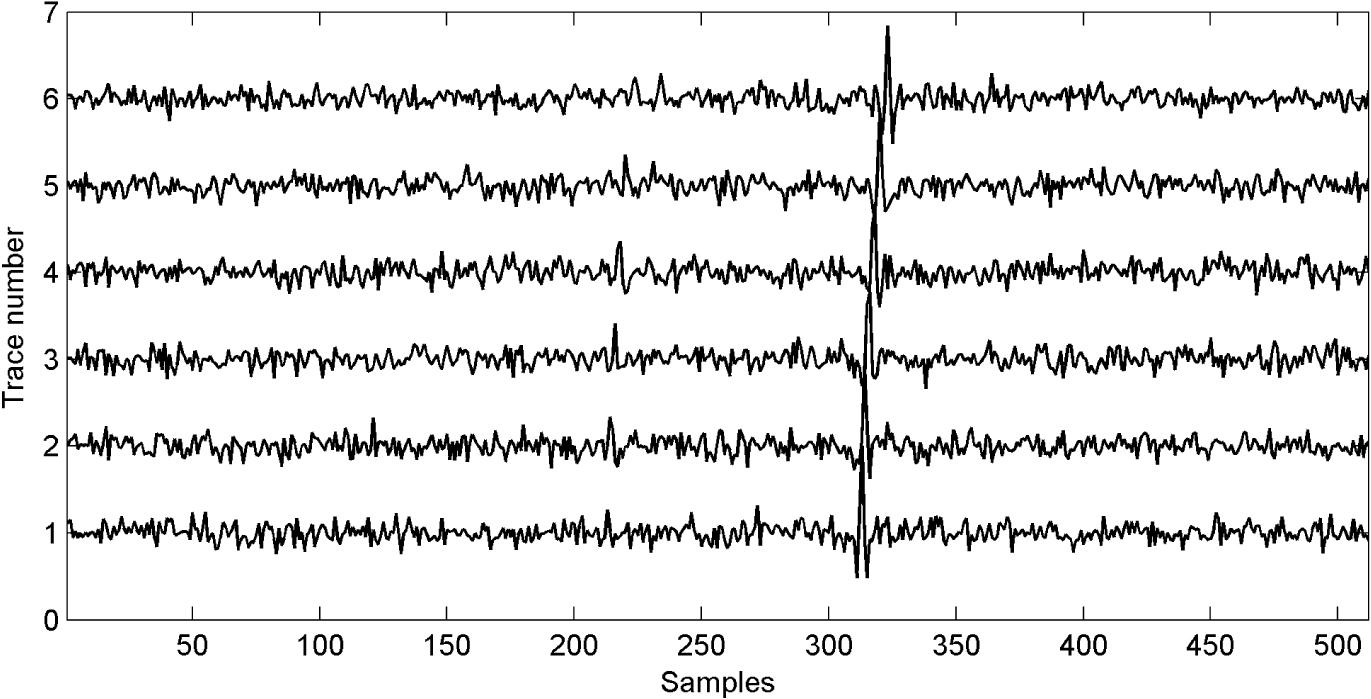Figure 2.Noisy record contaminated by WGN with the SNR of −8 dB.

The record is decomposed into 4 scales by the shearlet transform. Since most microseismic signals concentrate on the high frequency scales, and the larger scales correspond to higher frequencies, we choose the larger scales for detection. In this paper, we divide records into 4 scales and we process the two largest scales: 3rd and 4th scale. Figure 3 shows the shearlet coefficients in different directions at the 3rd scale. We can see that most signals are concentrated in the 4th direction, while noise is distributed in all directions. The correlation energy of the first trace between adjacent scales is shown in Figure 4. We can see that the correlation energy in the 4th direction is obviously larger than the others. This indicates that we can obtain more accurate signal directions through correlation processing. Figure 5(a) shows the waveform of the first trace. We can see that the front low-amplitude microseismic signal is submerged by noise which is difficult to identify. The shearlet coefficients after the correlation processing is shown in Figure 5(b), the shearlet coefficients associated with microseismic signals are all distinguished from the noise, which facilitates the subsequent energy entropy feature extraction and SVM detection.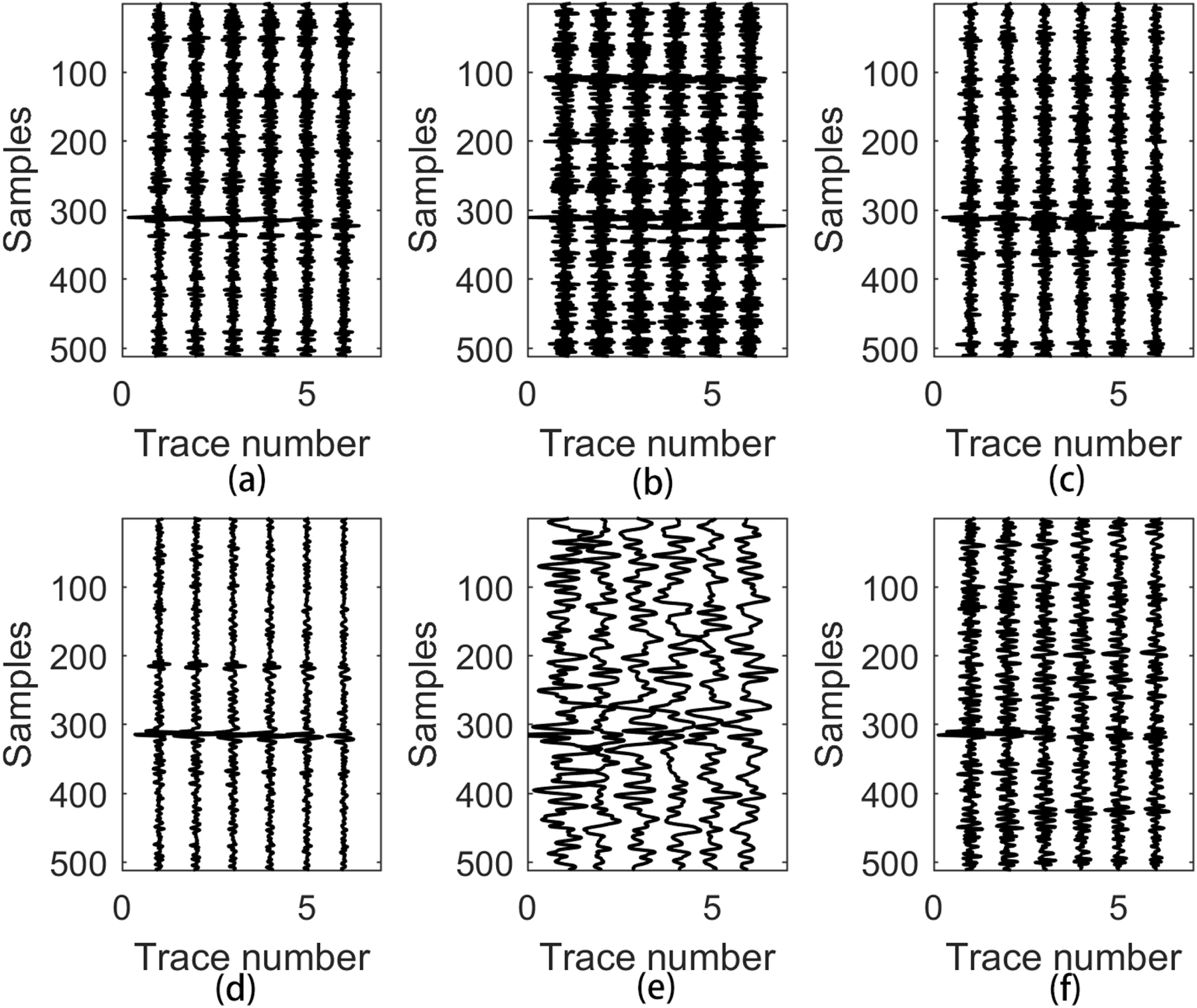Figure 3.(a)–(f) Correspond to the shearlet coefficients from the first to the sixth directions at the 3rd scale.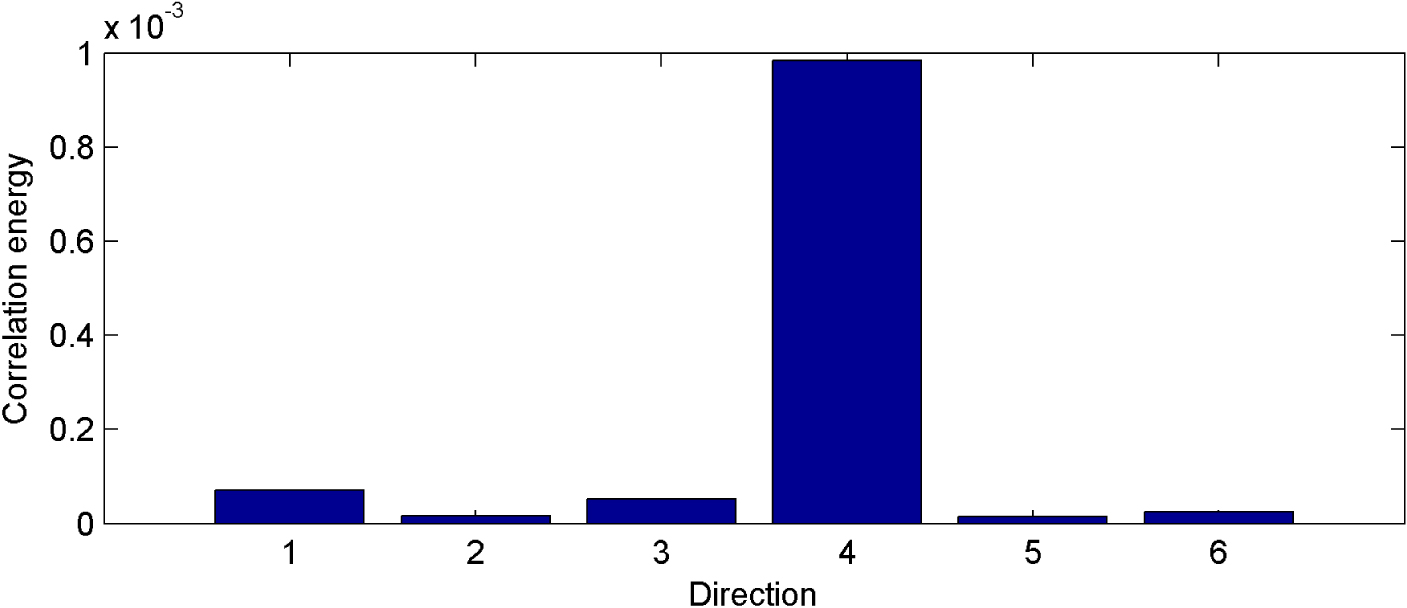Figure 4.The correlation energy of first trace between adjacent scales (j = 3 and j = 4).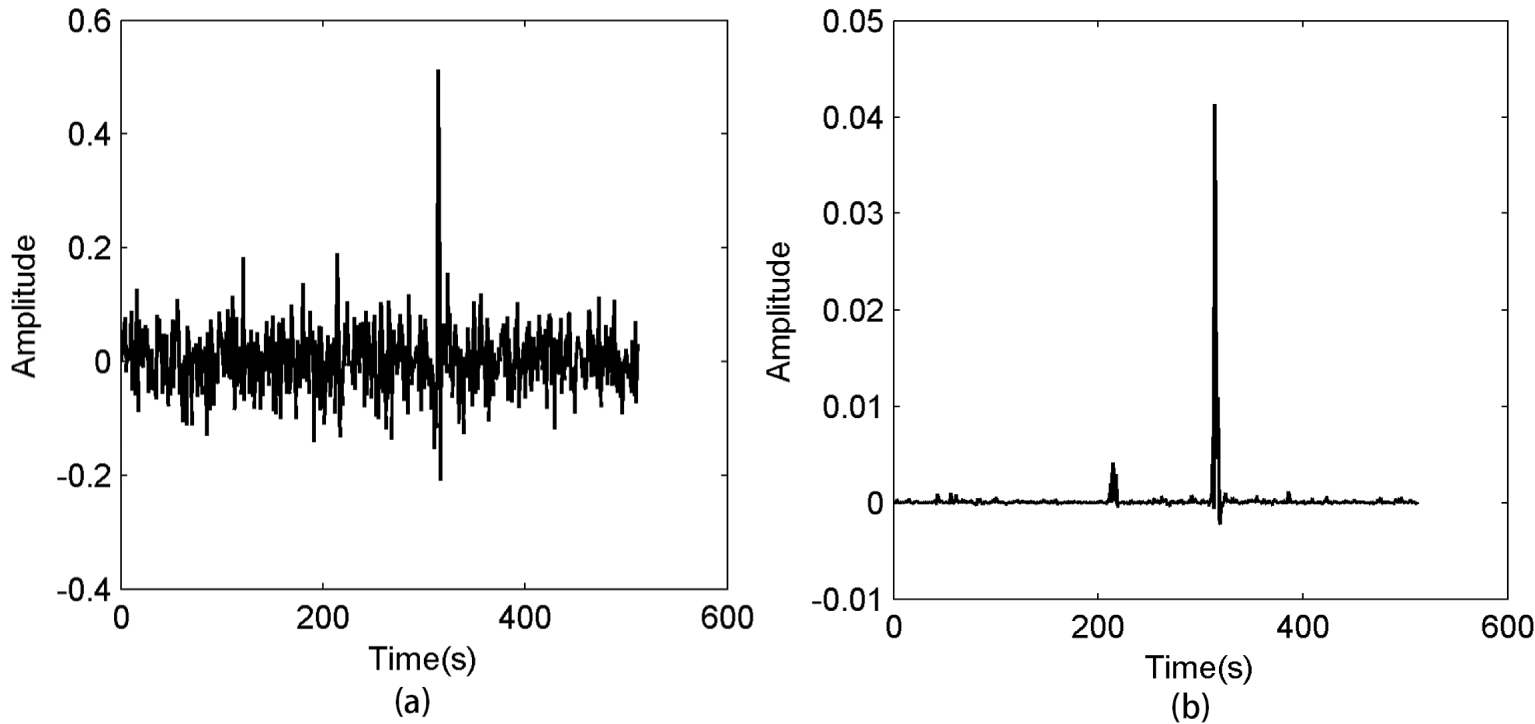Figure 5.(a) The waveform of the first trace from Figure 2. (b) Shearlet coefficients of the first trace after correlation processing.

Next comes the training process of the SVM. For the training set of the SVM classifier, we chose here 1000 groups of signal samples and 1000 groups of noise samples to form the training set. The signal samples are were randomly generated by using Ricker wavelets with the amplitudes ranging from 0.1 to 1 and frequencies ranging from 100 to 500 Hz. The noise samples were randomly generated with WGN and real noise with different levels. Through training, we can obtain the trained SVM classifier.

We compared the proposed method with the STA/LTA and CNN, and the detection results are shown in Figure 6. The proposed method can accurately detect two microseismic signals and there is no misjudgment. In the case of low SNR, we can still accurately detect the microseismic signals, while there are many detection errors with the STA/LTA and CNN methods. It is difficult to choose a suitable threshold for the STA/LTA method especially when the SNR is low. CNN achieves accurate detection at high SNR, but it does not work well for low SNR either. In contrast, the proposed method avoids threshold setting and the detection result of the proposed method is accurate at low SNR.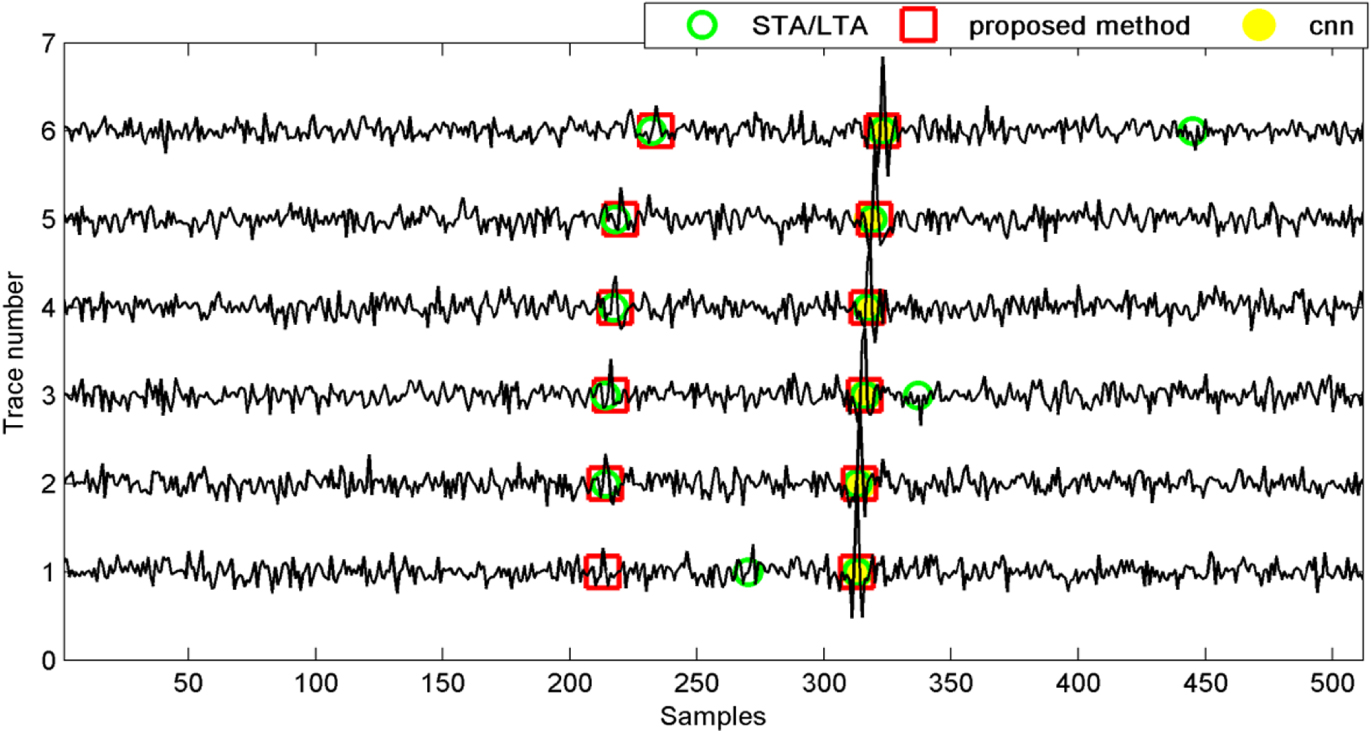Figure 6.Detection results of Figure 2 by the proposed method, STA/LTA and CNN.

### 4.2. Statistical experiments

In order to verify the validity of the proposed method, we pick 500 groups of samples to make the experiment. A Ricker wavelet is used to simulate the signal. The dominant frequency of Ricker wavelets is 200 Hz. The sampling frequency is 1000 Hz. We add different WGNs to pure Ricker wavelets and change the amplitudes of the Ricker wavelets. The detection results of the proposed method, STA/LTA and CNN are listed in Tables 12 and 3, respectively.

Table 1.

Accuracy of synthetic signal detection by the proposed method

AmplitudeSNR
−5 dB−6 dB−7 dB−8 dB
1 100%99.2%92.4%87%
0.5 100%98.6%92.2%85%
0.2 99.8%98.2%91.4%80%

Table 2.

Accuracy of synthetic signal detection by STA/LTA

AmplitudeSNR
−5 dB−6 dB−7 dB−8 dB
1 97%90.2%86.4%70%
0.5 90%89.5%85.6%69%
0.2 87%87%83.4%63.4%

Table 3.

Accuracy of synthetic signal detection by CNN

AmplitudeSNR
−5 dB−6 dB−7 dB−8 dB
1 97.8%94%89%76%
0.5 92.5%89.6%85%73.1%
0.2 91%88%84.6%68.2%

From Tables 12 and 3, we can see that the detection accuracy decreases as the SNR and signal amplitude decrease. The proposed method achieves higher accuracy at low SNR compared with the STA/LTA and CNN. The accuracy of the proposed method is 80% when the SNR is −8 dB. The accuracy of STA/LTA and CNN is only 60%–76%. We draw receiver operating characteristic (ROC) curves of 100 groups of samples when SNR is −8 dB and the amplitude of the Ricker wavelet is 0.2 as shown in Figure 7. A method works more accurately if its ROC curve is closer to the upper left corner. The AUC is the value of the area under the ROC curve which is used to conclude whether a classifier is excellent. When an AUC value of a method is larger, this method has higher accuracy. The AUC values of the proposed methods, STA/LTA and CNN, are 0.8570, 0.7091 and 0.7536, respectively. As shown by Figure 7, the ROC curve of the proposed method is closer to the upper left upper corner and its AUC value is larger than that of the other two methods. Thus the proposed method has higher accuracy than the other two methods. To further verify the effectiveness of the proposed method, we add real noise with different levels to the above synthetic microseismic signals to compose 300 group samples: the amplitude of synthetic microseismic signals is 1 in the first 100 groups, 0.5 in the second 100 groups, and 0.2 in the last 100 groups. The detection results of the proposed method, STA/LTA and CNN are listed in Table 4. We can see that the proposed method still has the highest accuracy in all cases.

Table 4.

Detection accuracy of synthetic signal contaminated with real microseismic noise

Amplitude 1 0.5 0.2
The proposed method100%95%82%
STA/LTA 93%71%60%
CNN95.6%77%70%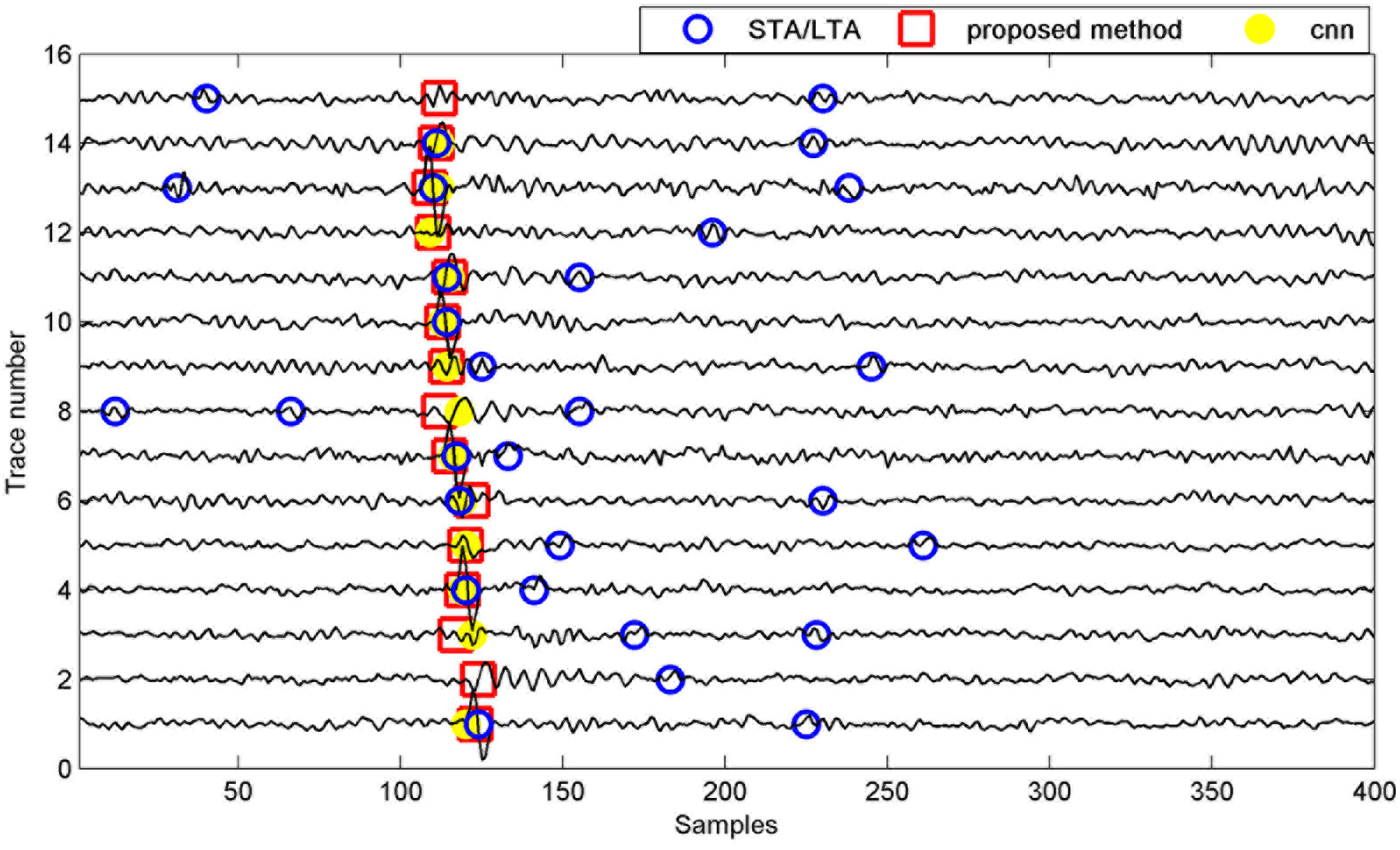Figure 9.Detection results of Figure 8 by the proposed method, STA/LTA and CNN.

### 4.3. Real microseismic and desert seismic data

In order to prove the validity of the proposed method in dealing with real data, we selected for analysis and processing a real microseismic record with 15 traces in a certain area of China, as shown in Figure 8 (this record is also used in some published papers such as Li et al. , Zhu et al. ). We can see that the amplitudes of signals in some traces are too weak to detect. These weak signals also have waveforms similar to those of the noise. The detection results of the proposed method, STA/LTA and CNN are shown in Figure 9. The STA/LTA method does not work well for this situation. Some noise with a similar amplitude and waveform is identified as a signal by the STA/LTA method. CNN identifies the low-amplitude signals as noise and thus they cannot be detected. High-frequency weak microseismic signal detection is very challenging for methods based on energy or deep learning. These weak signals can be accurately detected by the proposed method which performs well in low SNR.

This challenge also appears in the desert record. The random noise in a desert seismic record is concentrated to the low frequency bands which often overlaps with the seismic signal. It causes great difficulty in desert seismic signal detection. Figure 10 shows a real desert record with 30 traces. We can see that desert noise has a low frequency and a waveform similar to that of the signal. In this example, we also compare the proposed method with the STA/LTA and CNN, and their detection results are shown in Figure 11. The performance of the STA/LTA dramatically decreases for weak desert seismic signal detection. Most signals can be detected by the CNN, but some noise sequences are also identified as signals. The proposed method has the highest accuracy compared with the STA/LTA and CNN.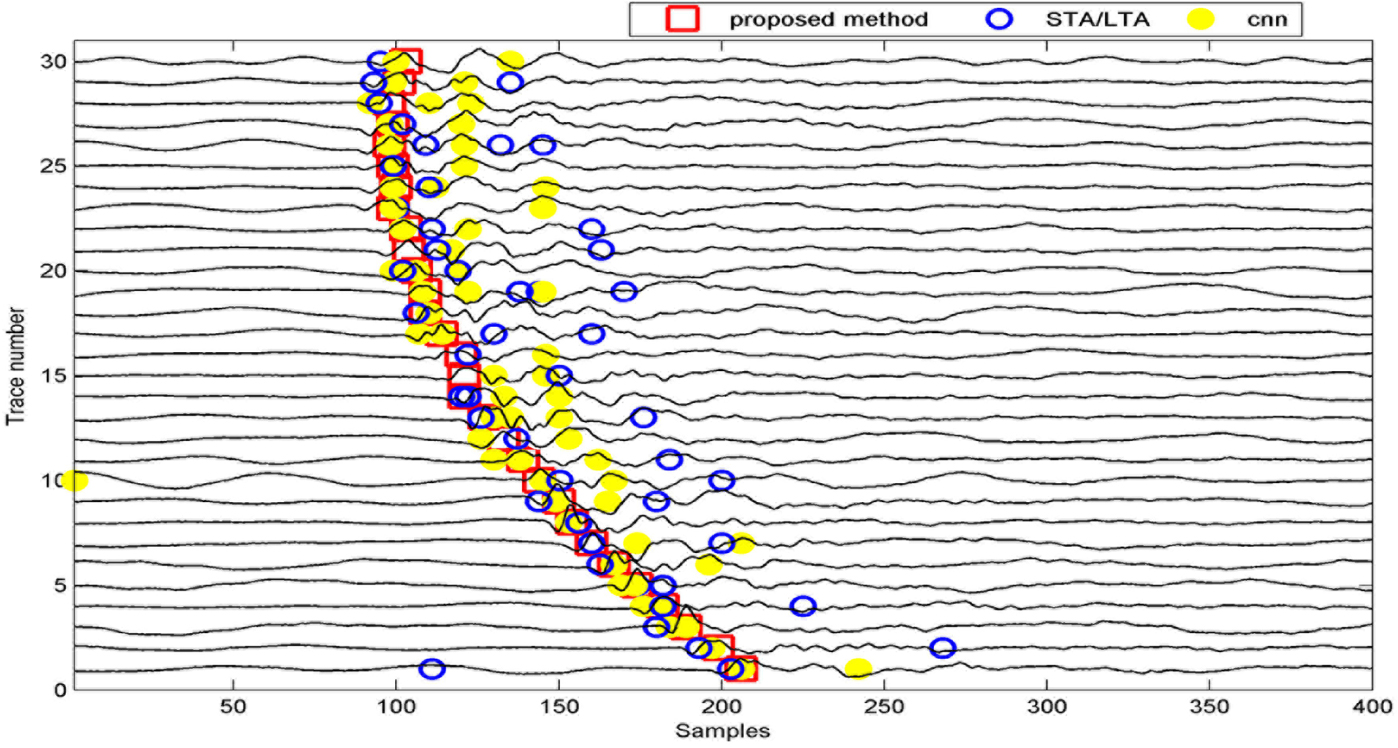Figure 11.Detection results of Figure 10 by the proposed method, STA/LTA and CNN.

## 5. Conclusion and discussion

In this paper, shearlet energy entropy is used as a signal feature to detect effective signals. This feature can better differentiate the signal from the noise than a simple energy computation. The SVM classifier is trained with the extracted feature. There is no need to select a reasonable signal detection threshold with the use of SVM. The detection errors due to inappropriate threshold selection can be reduced. The signal is detected in an intelligent way by the proposed method. A large number of experiments demonstrate the potential and superiority of this proposed method for low-amplitude microseismic and seismic signal detection. It requires much fewer training datasets than the CNN- based signal detection methods.

In the proposed method, shearlet energy entropy is used as a feature to train a SVM classifier for signal detection. A more effective classifier can be obtained by combining more features. In addition, a more refined kernel-function selection in the SVM such as a mixed-kernel function can further increase the classification accuracy.

## Acknowledgements

This research is financially supported by the National Natural Science Foundations of China (under grants 41730422, 41974143, 41574096). We thank the efforts made by Professor Ghislain de Marsily. We also thank the anonymous reviewers for their comments and suggestions.

References

[Adankon and Cheriet, 2009] M. M. Adankon; M. Cheriet Model selection for the LS-SVM. Application to handwriting recognition, Pattern Recognit., Volume 42 (2009) no. 12, pp. 3264-3270 | Article

[Allen, 1982] R. V. Allen Automatic phase pickers their present use and future prospects, Bull. Seismol. Soc. Am., Volume 72 (1982) no. 6, pp. 225-242 | Article

[Easley et al., 2008] Q. Easley; D. Labate; W. Q. Lim Sparse directional image representations using the discrete Shearlet transform, Appl. Comput. Harmonic Anal., Volume 25 (2008) no. 1, pp. 25-46 | Article | MR: 2419703 | Zbl: 1147.68794

[Gao et al., 2018] D. W. Gao; Z. H. Zhao; H. Zhang Micro-seismic real-time monitoring technology for staged fractudng wells in Jiaoshiba Block, Well Test., Volume 27 (2018) no. 5, pp. 42-49 | Article

[Guo and Labate, 2006] K. Guo; D. Labate The theory of wavelets with composite dilations, Harmon. Anal. Appl., Volume 10 (2006), pp. 231-318 | Article | Zbl: 1129.42433

[Guo and Labate, 2007] K. Guo; D. Labate Optimally sparse multidimensional representation using the discrete shearlet transform, SIAM J. Math. Anal., Volume 39 (2007) no. 1, pp. 298-318 | Article

[Hu et al., 2013] R. H. Hu; P. H. Lou; D. B. Tang Fault diagnosis of rolling bearings based on EMD and parameter adaptive support vector machine, Comput.-Integr. Manuf. Syst., Volume 19 (2013) no. 2, pp. 438-447 | Article

[Jia et al., 2016] R. S. Jia; H. M. Sun; Y. J. Peng; Y. Q. Liang; X. M. Lu Automatic event detection in low SNR microseismic signals based on multi-scale permutation entropy and a support vector machine, J. Seismol., Volume 21 (2016) no. 4, pp. 1-14 | Article

[Leonard, 2000] M. Leonard Comparison of manual and automatic onset timepicking, Bull. Seismol. Soc. Am., Volume 90 (2000), pp. 1384-1390 | Article

[Li and Li, 2016] G. H. Li; Y. Li Random noise of seismic exploration in desert modeling and its applying in noise attenuation, Chin. J. Geophys., Volume 59 (2016) no. 2, pp. 682-692 | Article

[Li et al., 2018] Y. Li; Y. Wang; H. B. Lin; T. Zhong First arrival time picking for microseismic data based on DWSW algorithm, J. Seismol., Volume 22 (2018) no. 4, pp. 833-840 | Article

[Lim, 2010] W. Q. Lim The discrete shearlet transform: a new directional transform and compactly supported shearlet frames, IEEE Trans. Image Process., Volume 19 (2010) no. 5, pp. 1166-1180 | Article | MR: 2723739 | Zbl: 1371.94233

[Mallat and Hwang, 1992] S. Mallat; W. L. Hwang Singularity detection and processing with wavelets, IEEE Trans. Inf. Theory, Volume 38 (1992), pp. 617-643 | Article | MR: 1162218 | Zbl: 0745.93073

[Maxwell and Urbancic, 2001] S. C. Maxwell; T. I. Urbancic The role of passive microseismic monitoring in the instrumented oil field, The Leading Edge, Volume 20 (2001), pp. 636-639 | Article

[Rezek and Roberts, 1998] I. A. Rezek; S. J. Roberts Stochastic complexity measures for physiological signal analysis, IEEE Trans. Biomed. Eng., Volume 45 (1998) no. 9, pp. 1186-1191 | Article

[Samaneh et al., 2018] R. P. Samaneh; M. Milos; P. Supathorn A novel framework for intelligent signal detection via artificial neural networks for cyclic voltammetry in pyroprocessing technology, Ann. Nucl. Energy, Volume 111 (2018), pp. 242-254

[Wang, 2009] Z. Wang Detection of weak earthquake signals based on wavelet analysis, Prog. Explor. Geophys., Volume 32 (2009) no. 3, pp. 182-185 | Article

[Widodo and Yang, 2007] A. Widodo; B. S. Yang Support vector machine in machine condition monitoring and fault diagnosis, Mech. Syst. Signal Process., Volume 21 (2007) no. 6, pp. 2560-2574 | Article

[Xiong et al., 2018] Z. H. Xiong; M. P. Nash; E. Cheng; V. V. Fedorov; M. K. Stiles; J. C. Zhao ECG signal classification for the detection of cardiac arrhythmias using a convolutional recurrent neural network, Physiol. Meas., Volume 39 (2018) no. 9, 094006 pages | Article

[Yuan et al., 2018] S. Yuan; J. Liu; S. Wang; T. Wang; P. Shi Seismic waveform classification and first-break picking using convolution neural networks, IEEE Geosci. Remote Sens. Lett., Volume 15 (2018) no. 2, pp. 272-276 | Article

[Zhang and Van der Baan, 2018a] C. Zhang; M. Van der Baan A denoising framework for microseismic and reflection seismic data based on block matching, Geophysics, Volume 83 (2018a) no. 5, p. V283-V292 | Article

[Zhang and Van der Baan, 2018b] C. Zhang; M. Van der Baan Multicomponent microseismic data denoising by 3D shearlet transform, Geophysics, Volume 83 (2018b) no. 3, p. A45-A51 | Article

[Zhang and Van der Baan, 2019] C. Zhang; M. Van der Baan Strong random noise attenuation by shearlet transform and time-frequency peak filtering, Geophysics, Volume 84 (2019) no. 6, p. V319-V331 | Article

[Zhang et al., 2004] X. G. Zhang; J. J. Xu; G. Y. Ge Defects recognition on X-ray images for weld inspection using SVM, Proceedings of 2004 International Conference on Machine Learning and Cybernetics, Volume 6, IEEE, 2004, pp. 3721-3725 | Article

[Zhao et al., 2016] X. Zhao; Y. Li; G. H. Zhuang; C. Zhang; X. Han 2-D TFPF based on Contourlet transform for seismic random noise attenuation, J. Appl. Geophys., Volume 129 (2016), pp. 158-166 | Article

[Zhu et al., 2016] D. Zhu; Y. Li; C. Zhang Automatic time picking for microseismic data based on a fuzzy C-means clustering algorithm, IEEE Geosci. Remote Sens. Lett., Volume 13 (2016) no. 12, pp. 1900-1904 | Article